### Home > PC > Chapter 11 > Lesson 11.1.3 > Problem11-47

11-47.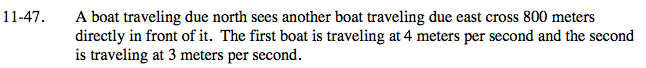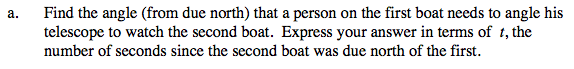Draw and label a diagram.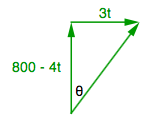Write an equation with θ and t. Use sine, cosine, or tangent.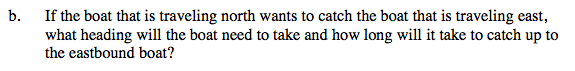Draw a new diagram. The first boat will now travel on the diagonal.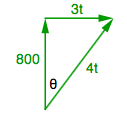Write equations with θ and t. Use sine, cosine, tangent and/or the Pythagorean theorem.